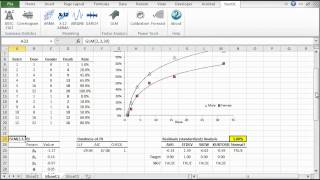# Generalized Linear Models (GLM)

The generalized linear model (GLM) is a flexible generalization of ordinary linear regression. By allowing the linear model to be related to the response variable via a link function, GLM in Excel generalizes linear regression. It also allows the magnitude of the variance of each measurement to be a function of its' predicted value.Upon completion, the GLM in Excel modeling function prints the selected model's parameters and tests/calculations in the designated location of your worksheet.